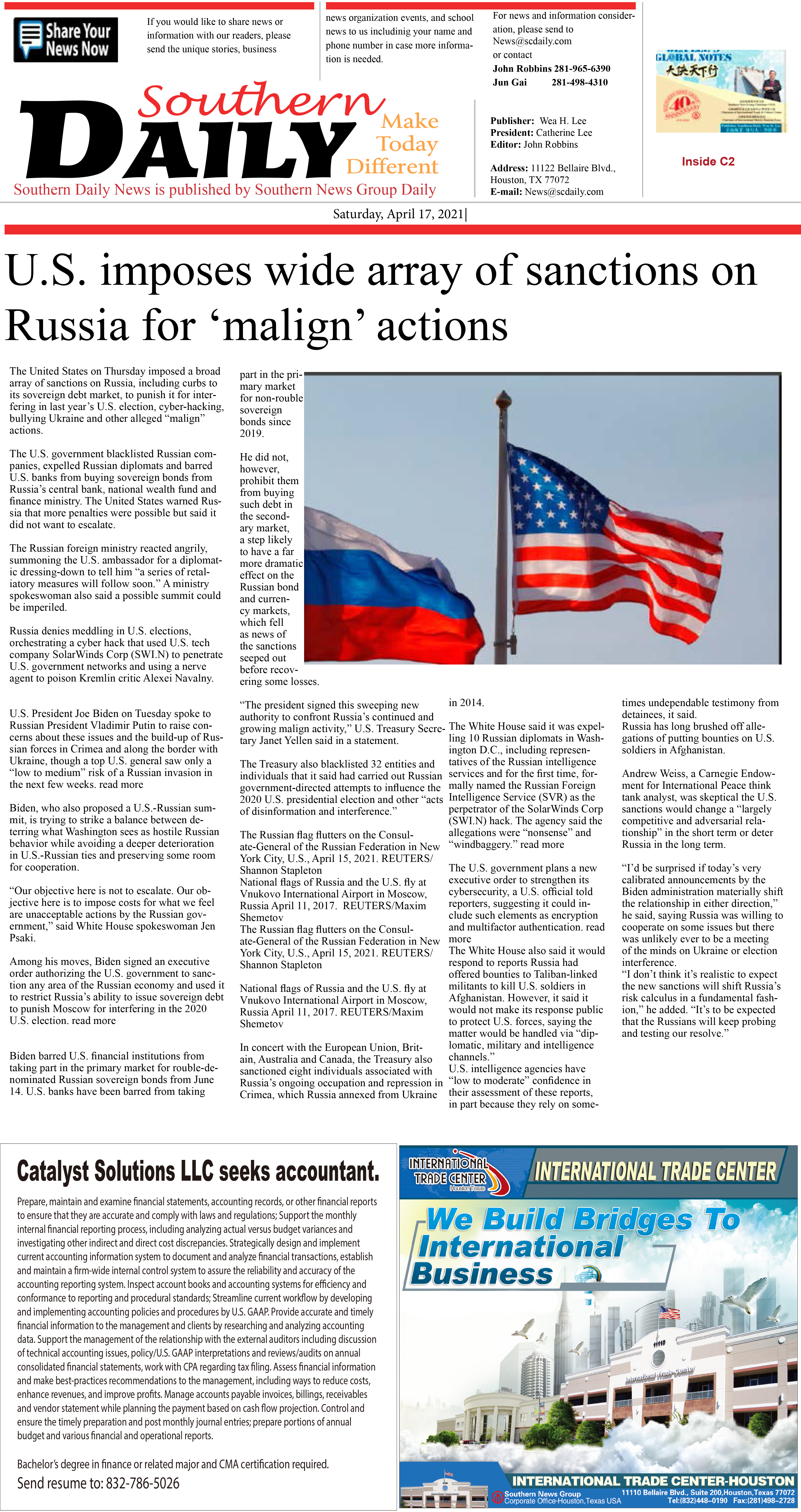210427 Epaper

 A Section B SectionC Section D Section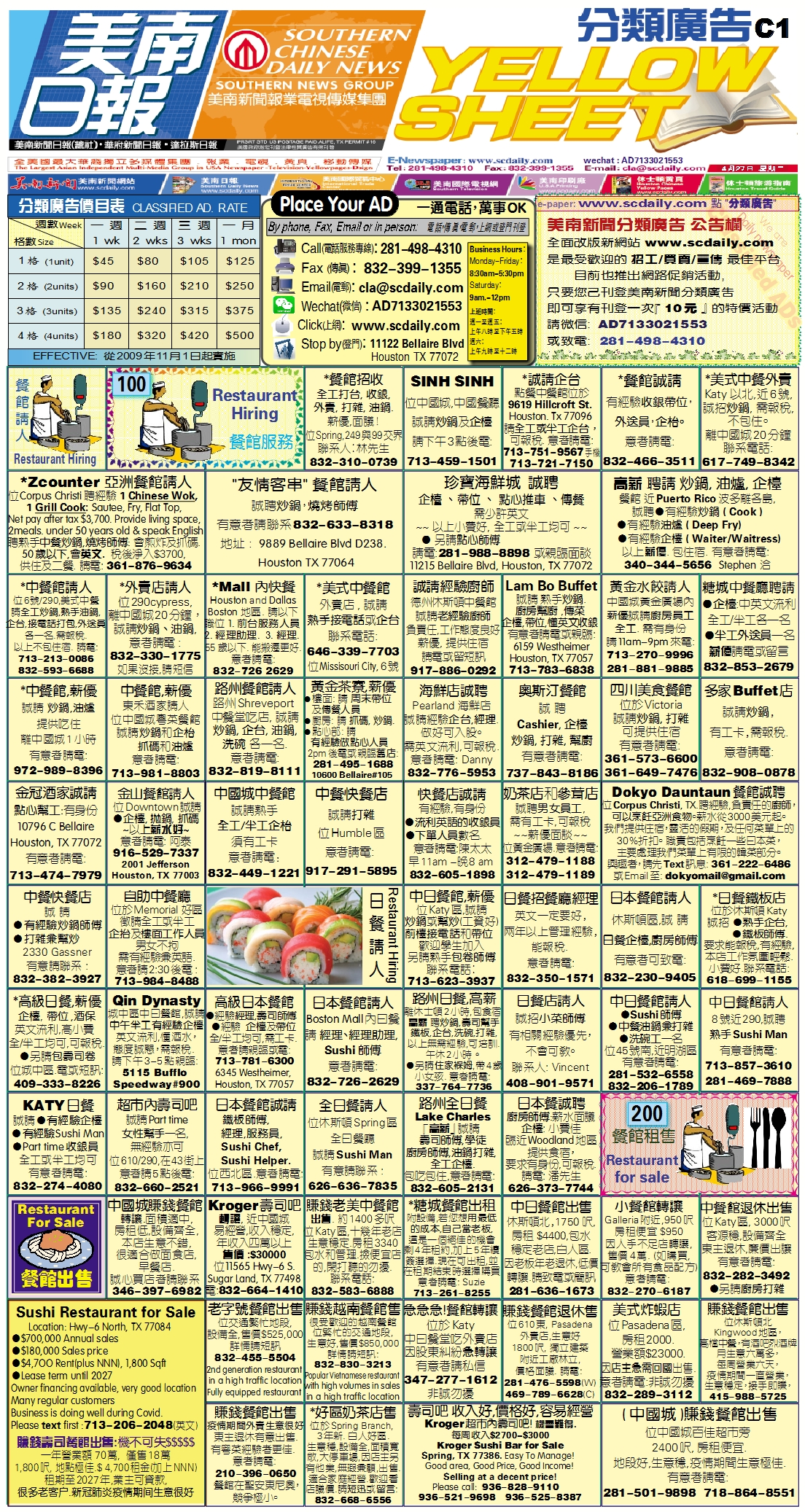210426 Epaper

 A Section B SectionC Section D Section210425 Epaper

 A Section B SectionC Section D Section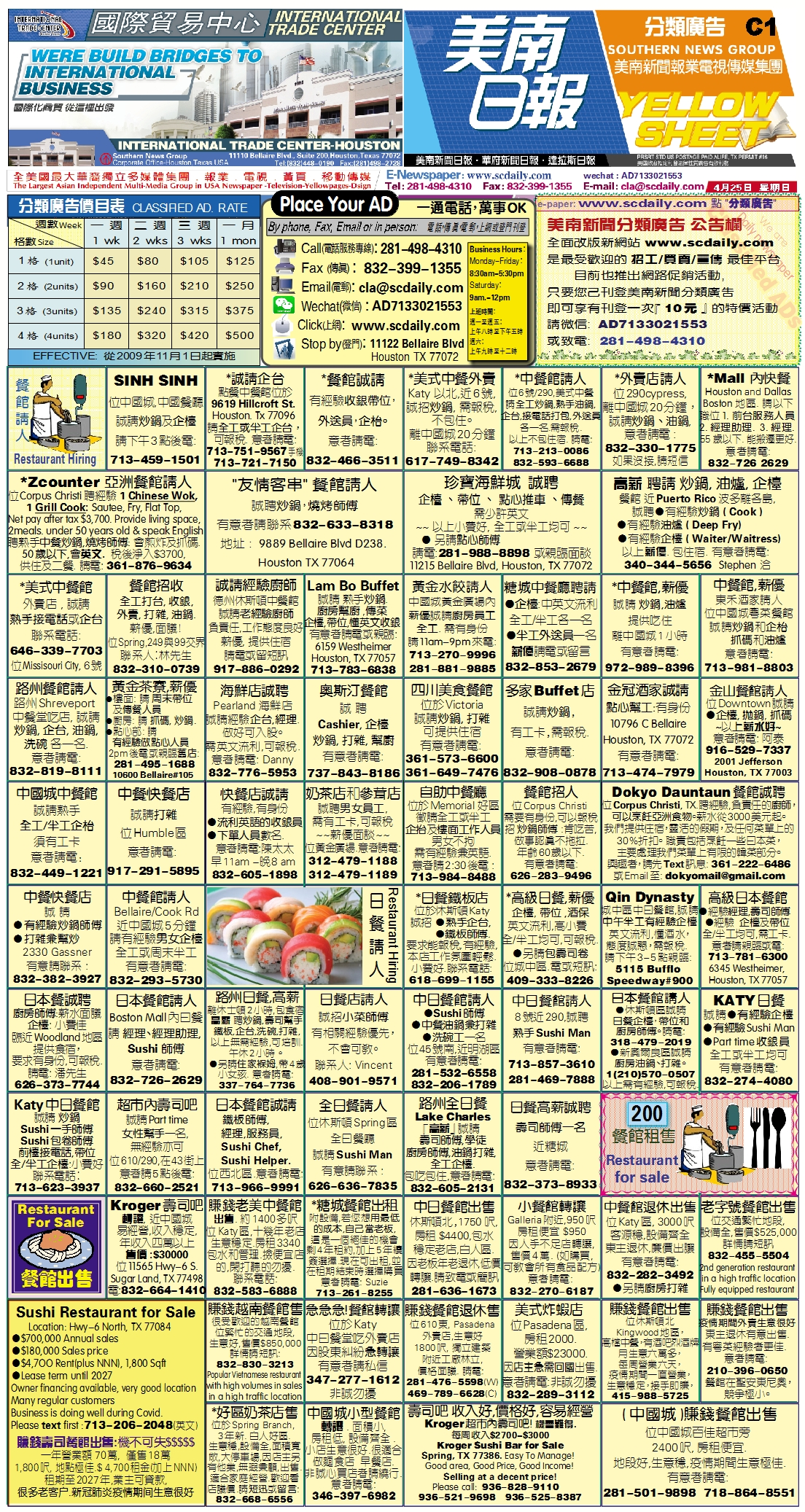210424 Epaper

 A Section B SectionC Section D Section210423 Epaper

 A Section B Section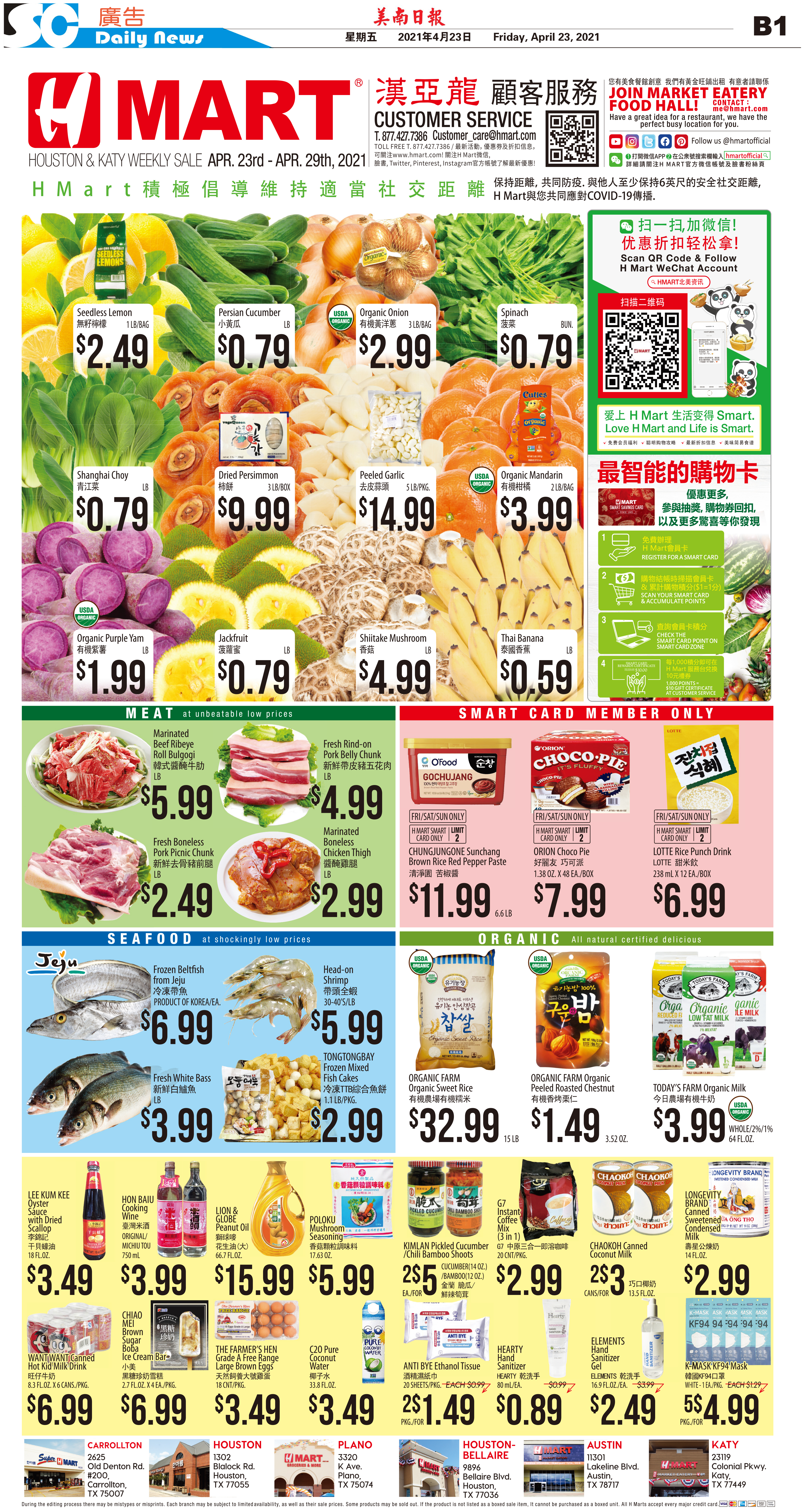C Section D Section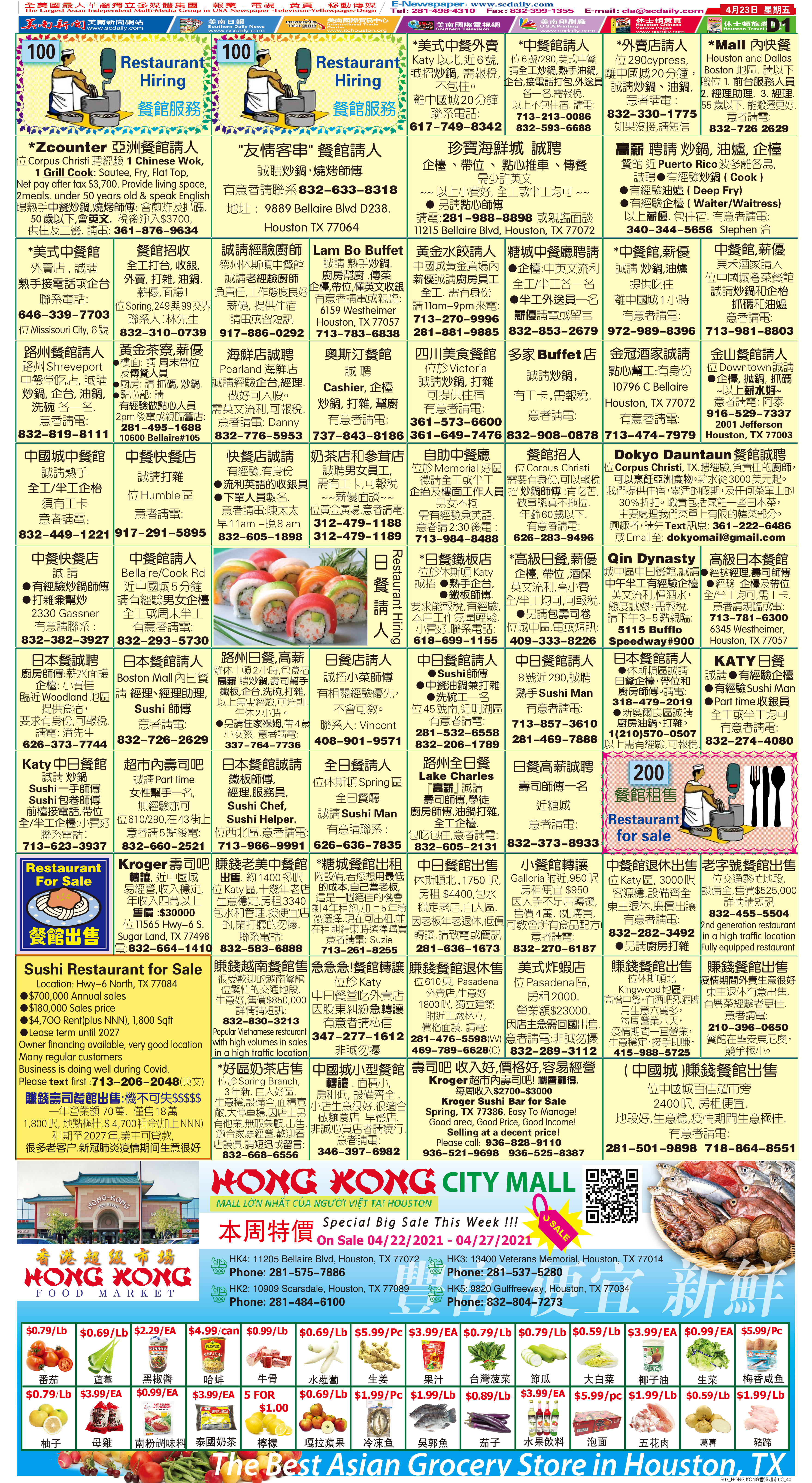210422 Epaper

 A Section B SectionC Section D Section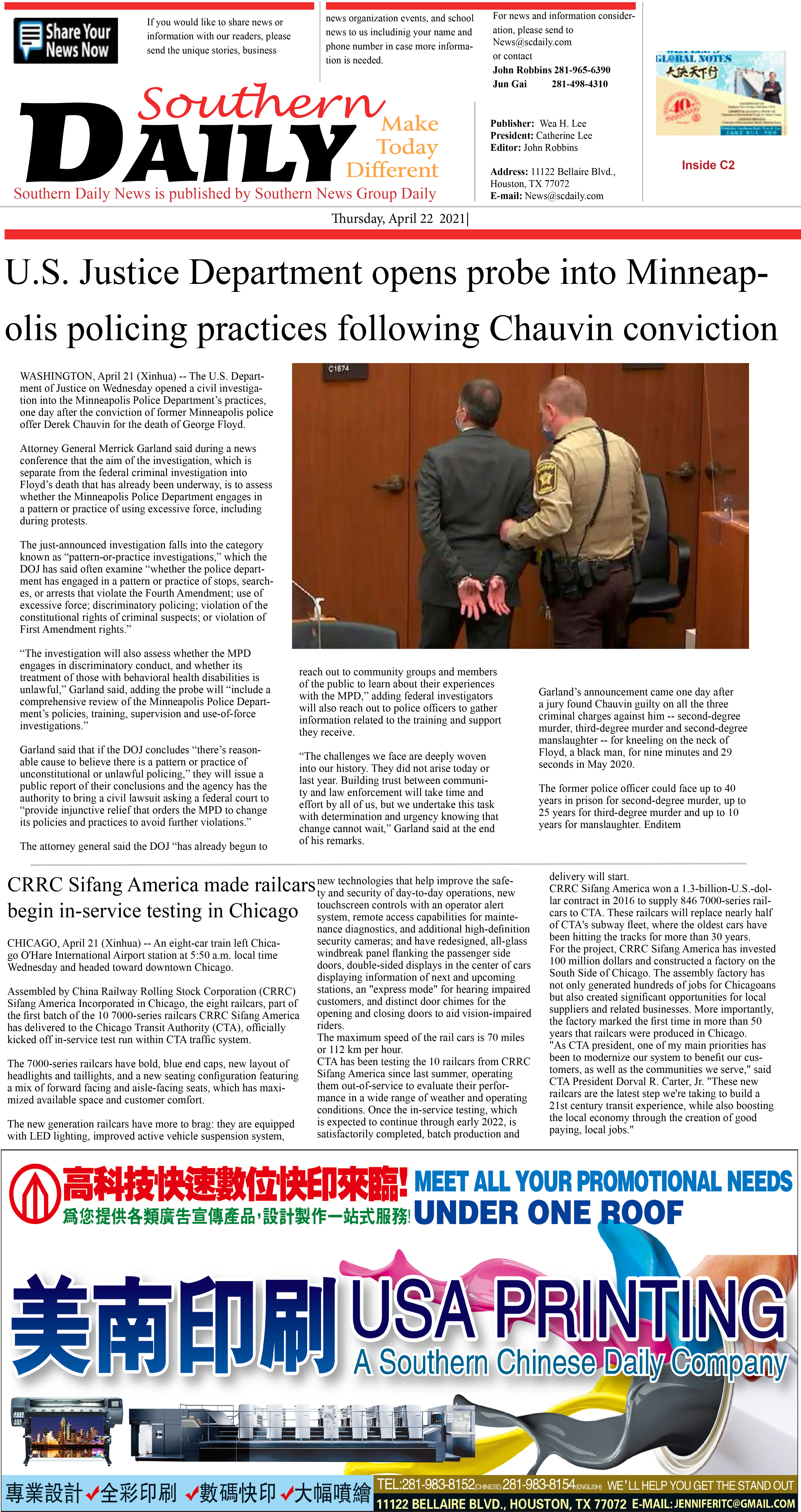210421 Epaper

 A Section B SectionC Section D Section210420 Epaper

 A Section B SectionC Section D Section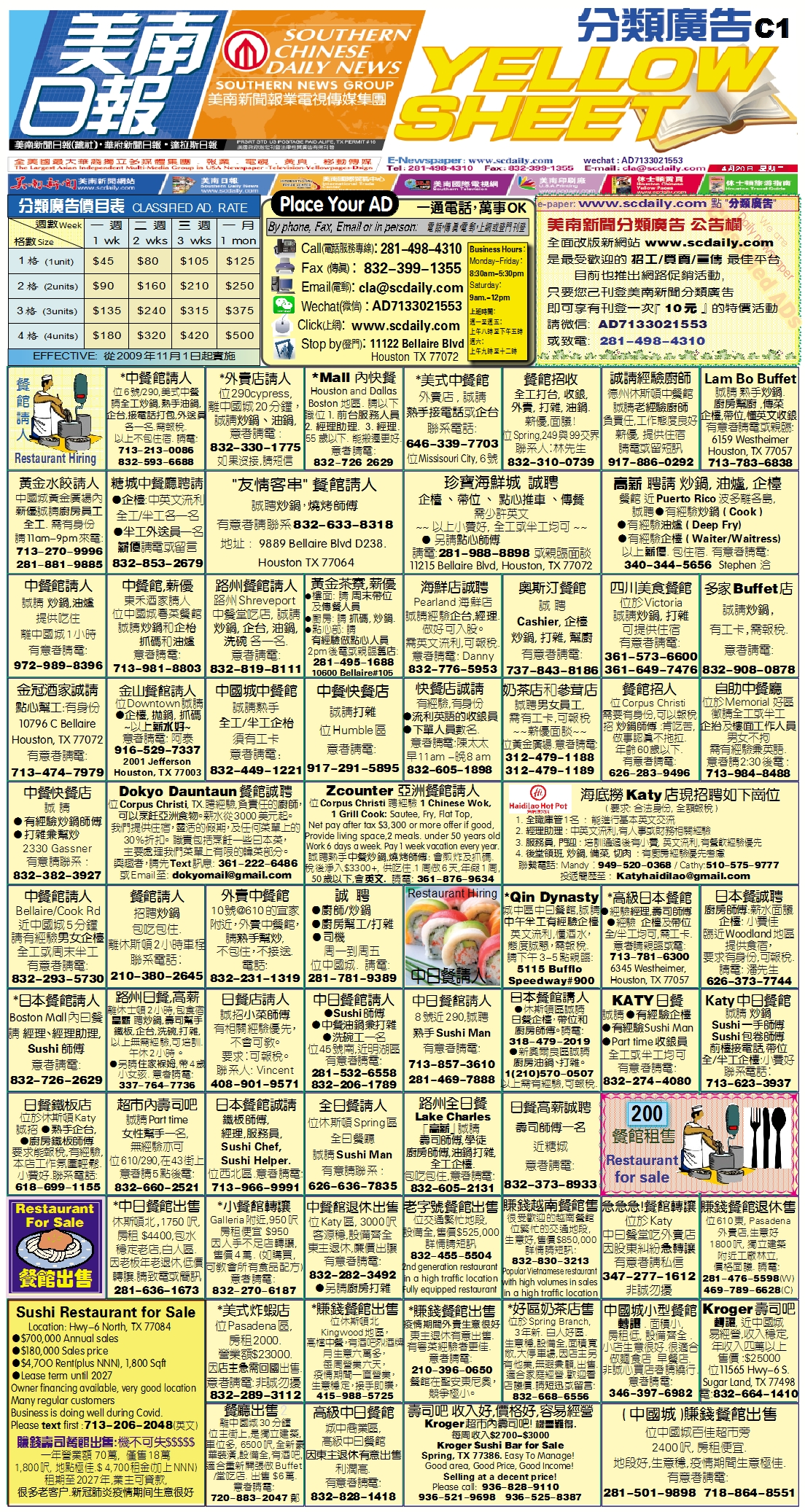210418 Epaper

 A Section B SectionC Section D Section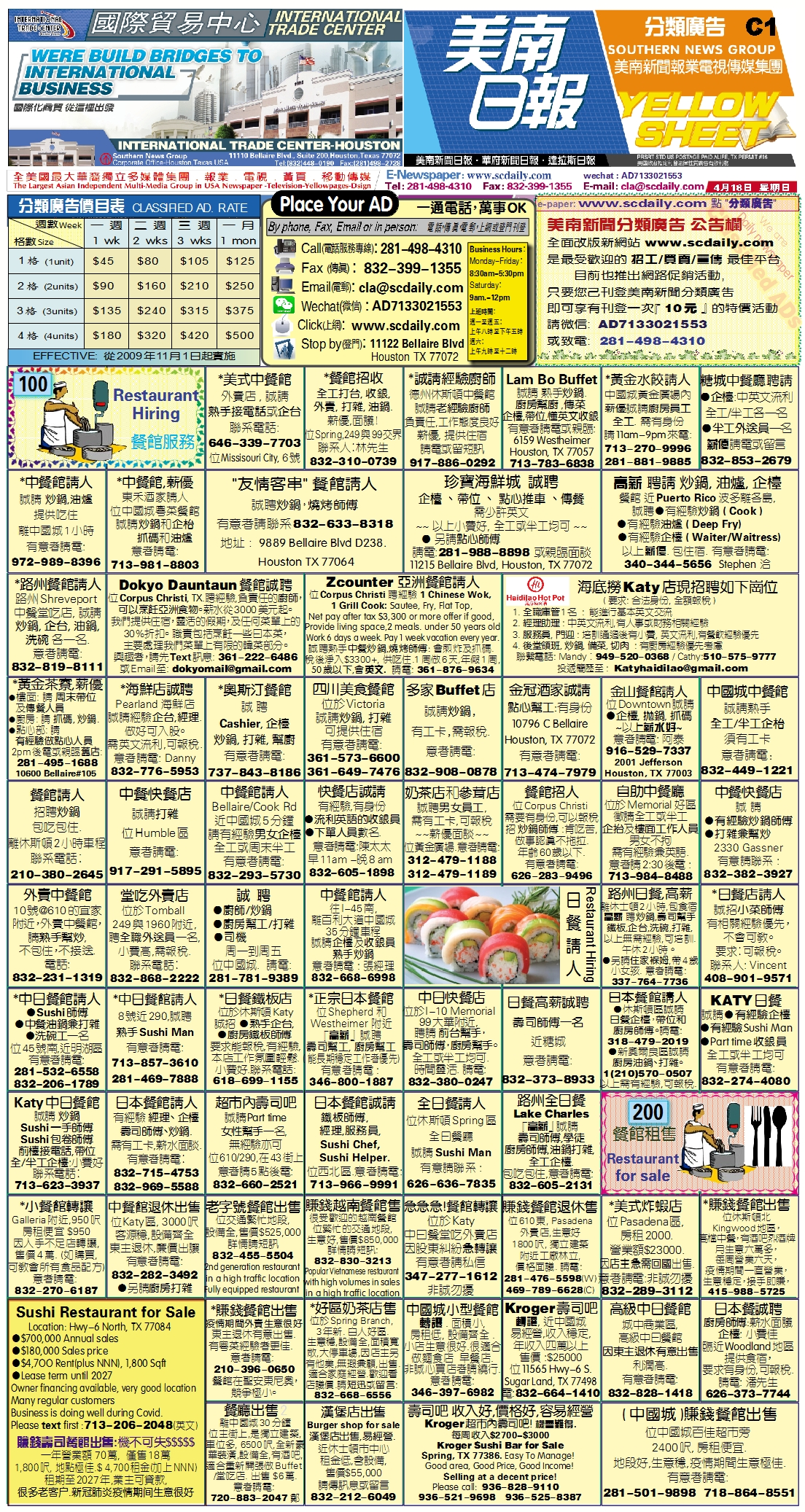210419 Epaper

 A Section B SectionC Section D Section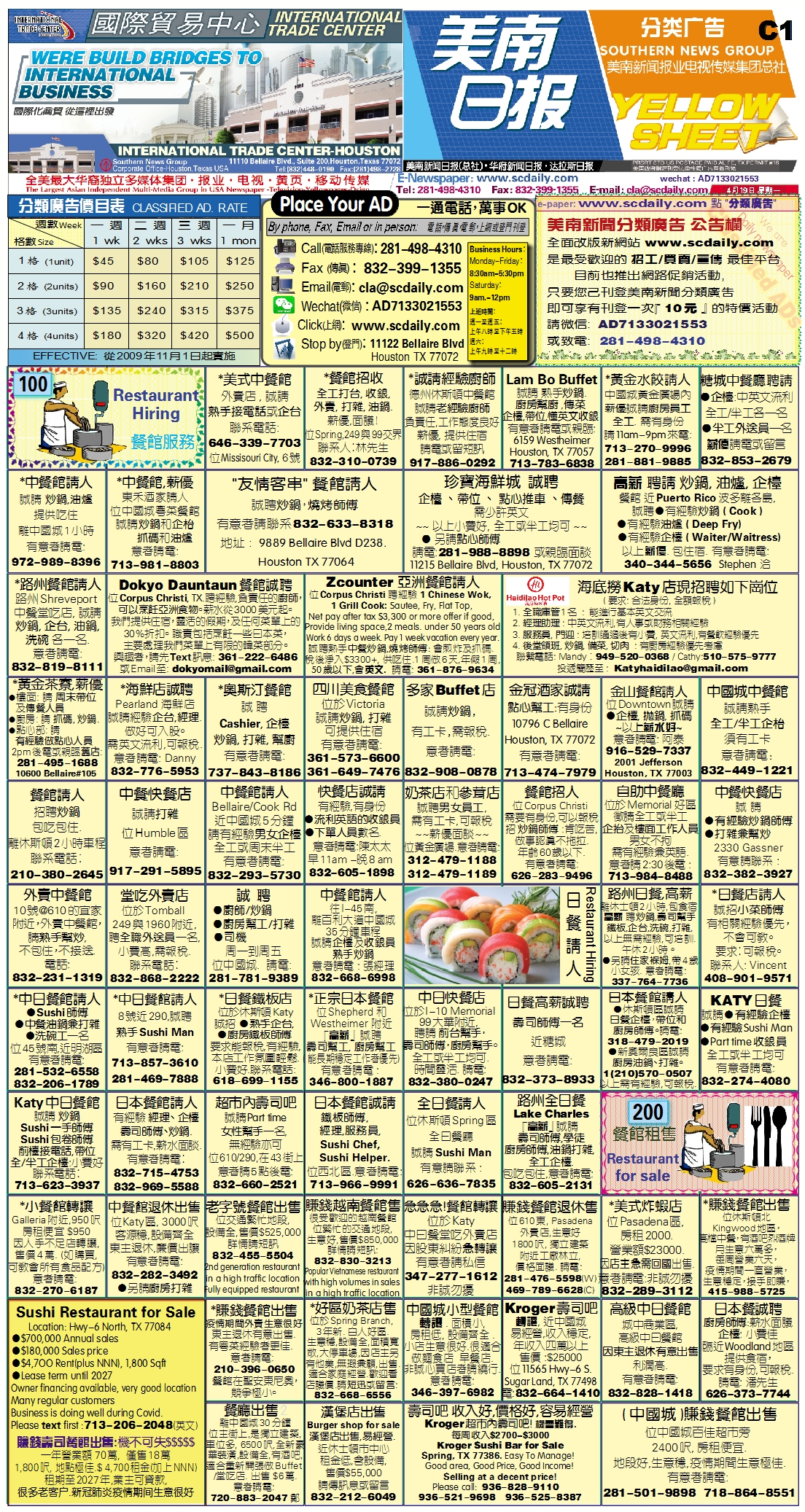210417 Epaper

 A Section B SectionC Section D Section